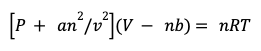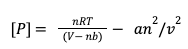Ideal Gases vs Real Gases – MCAT General Chemistry | MedSchoolCoach

## Sample MCAT Question

Which of the following variables are used to correct for volume in the Van der Waals equation for real gases?

a) Moles of gas

b) Strength of intermolecular forces

c) Concentration of gas

d) Temperature

A is correct. Moles of Gas. In order to correct the ideal gas law for real gases, the volume is subtracted by the product of moles of gas and volume of gas particles. This corrects for the ideal gas law assumption that gas particles have negligible volume by subtracting the overall volume of all of the gas particles. None of the other choices like strength of intermolecular forces, concentration, or temperature are relevant to correcting the volume.## Ideal Gases vs Real Gases

Ideal gases do not exist in nature, but real gases approximate models of ideal gases under conditions of high temperature and low pressure. However, under conditions of low temperatures and/or high pressures, a real gas can no longer be treated as an ideal gas. In those cases, we need to have an understanding of how real gases behave. To understand the differences between ideal gases and real gases, we’ll compare the volume and pressure of each.

## Volume

When considering ideal gases, the volume they occupy is simply the volume of the container. This is true because we assume that ideal gas particles have negligible volume. In contrast, real gases take up space, so the volume they occupy is less than the total volume of the container. The volume available for a real gas particle is equal to the total volume of the container minus the volume occupied by molecules of gas.The ideal gas law equation (PV = nRT) doesn’t account for the reduced volume available to molecules of a real gas. Here is the ideal gas law with the corrections accounting for the volume of real gases shown, set equal to pressure:For the volume available to real gases, you have to subtract the volume occupied by the gas molecules, equal to the product of n times b. The number of moles of gas is n, while b can be thought of as a constant that describes how large the molecules are. When you subtract the product of these two, you’re able to subtract the volume occupied by the gas particles, correcting for the volume.

## Pressure

With ideal gases, the gas particles move unencumbered and freely collide with the walls of the container to produce the pressure. This is true because ideal gas molecules do not experience intermolecular forces, as one of our assumptions for ideal gases states.

Conversely, real gas molecules do experience attractive intermolecular forces from one another, such that the gas particles don’t move independent of one another. Consequently, the gas molecules have a tendency to clump in the middle and don’t collide as frequently with the walls of the container. Therefore, real gases produce a lower pressure than ideal gases. Let’s revisit the Van Deer Waal’s equation, and how it corrects for the pressure of real gases. Below is our equation with corrections for volume, minus a new term.The variable a is a constant representing the strength of the attractive forces. The variable n represents moles of gas, while v represents the volume. The relationship “n over v” is moles per volume, which expresses the concentration of gas particles in a container. The greater the concentration of gas particles, the more intermolecular interactions the gas molecules will have. A greater number of intermolecular interactions will lead to a reduced number of collisions, decreasing the total pressure. Stronger and more numerous intermolecular interactions will decrease the pressure of real gases, so an2/v2 is the correction for pressure. This equation can be re-written into the typical form of the Van der Waals equation for real gases.It is not recommended that you memorize the equations shown here. Instead, make sure you’re able to understand why we corrected for the volume as well as why we corrected from the pressure for real gases in comparison to ideal gases.

### MCAT Go

Engaging audio learning to take your MCAT learning on the go, any time, any where. You'll be on the way to a higher MCAT score no matter where you are. Listen to over 200+ lessons.

### MCAT Practice Exams

Practice makes perfect! Our representative exams coupled with thorough explanations and in-depth analytics help students understand exactly where they stand.

### One-on-One Tutoring

Are you ready to take your MCAT performance to a whole new level? Work with our 99th-percentile MCAT tutors to boost your score by 12 points or more!